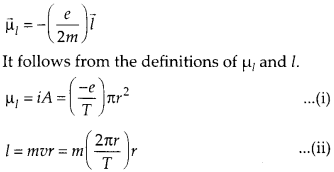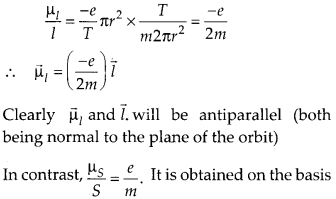Enlightened

# Question 25: NCERT Solutions for 12th Class Physics: Chapter 5-Magnetism and Matter

• 0

Question 25: NCERT Solutions for 12th Class Physics: Chapter 5-Magnetism and Matter

The magnetic moment vectorsand

associated with the intrinsic spin angular momentumand orbital angular momentumrespectively, of an electron are predicted by quantum theory (and verified experimentally to a high accuracy) to be given by=and=Which of these relations is in accordance with the result expected classically? Outline the derivation of the classical result.

Share

1. Solution:
Out of the two relations given, only one is in accordance with classical physics. This iswhere r is the radius of the circular orbit, which the electron of mass m and charge (- e) completes in time T. Divide (i) by (ii),of quantum mechanics.

Check the complete chapter with solutions.

NCERT Solutions for 12th Class Physics: Chapter 5-Magnetism and Matter

• 0